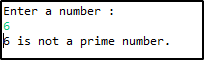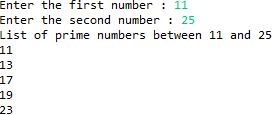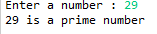# Know all about the Prime Number program in Java15 / 29 Blog from Java Programs

A prime number is a natural number greater than 1 which are divisible by only 1 and itself. For example 2, 3, 5, 7, 11…  are prime numbers because they can neither be divided nor is a result of the multiplication. Programs on Prime numbers are one of the most frequently asked Java interview questions for freshers. In this post, I have collected some of the important prime number programs in Java.

## Program to check whether the given number is prime or not?

In this java program, I will take a number variable and check whether the number is prime or not.

• The isPrime(int n) method is used to check whether the parameter passed to it is a prime number or not. If the parameter passed is prime, then it returns True otherwise it returns False.
• If the number is less than 1, if(inputNumber<= 1) it returns false.
• If the number is not less than or equal to 1, performs division operation.
• If the remainder is zero, it returns false, meaning it is not a prime number.
• If it is a non-zero number, it returns true, resulting in a prime number.
```package prime;

import java.util.Scanner;

{
static boolean checkForPrime(int inputNumber)
{
boolean isItPrime = true;

if(inputNumber <= 1)
{
isItPrime = false;

return isItPrime;
}
else
{
for (int i = 2; i<= inputNumber/2; i++)
{
if ((inputNumber % i) == 0)
{
isItPrime = false;

break;
}
}

return isItPrime;
}
}

public static void main(String[] args)
{
Scanner sc = new Scanner(System.in);

System.out.println("Enter a number :");

int inputNumber = sc.nextInt();

boolean isItPrime = checkForPrime(inputNumber);

if (isItPrime)
{
System.out.println(inputNumber+" is a prime number.");
}
else
{
System.out.println(inputNumber+" is not a prime number.");
}

sc.close();
}
}

```

Note: 0 and 1 are not prime numbers.

The output of this program is:Let us move to the next program to check prime number program in Java.

## Program to find out all prime number between two given numbers

To find the prime number between two natural numbers,

• Check if the number is a natural number.
• Use the IsPrime method to check if the number is prime or not.
• Specify the start number and also the end number.
• For loop to print the prime number.
• You can do that for a series of numbers just specify the range(start and end).
```import java.util.Scanner;

{
static boolean checkForPrime(int inputNumber)
{
boolean isItPrime = true;

if(inputNumber<= 1)
{
isItPrime = false;
return isItPrime;
}
else
{
for (int i = 2; i= inputNumber/2; i++){
if ((inputNumber % i) == 0){
IsItPrime = false;
break;
}
}
return isItPrime;
}
}
public static void main(String[] args)
{
Scanner sc = new Scanner(System.in);
System.out.println("Enter the start value :");
int start = sc.nextInt();
System.out.println("Enter the end value :");
int end = sc.nextInt();
System.out.println("Prime numbers between "+start+" and "+end+" : ");
for (int i = start; i <= end; i++)
{
if(checkForPrime(i))
{
System.out.println(i);
}
}
sc.close();
}
}
```

Output:Let’s move ahead to our next program to check prime number program in Java.

## Program to check whether the number is prime or not using recursion

• In this case, let’s use recursion to print the prime numbers.
• The Scanner class is a class which is present inside the java. util package, that allows the user to read values of various types.
• First check if the number is a natural number using if condition, if (n <= 1), return false and print that the number is not a prime number.
• Check for another condition, that is division, check if the remainder is 0 or not. If the remainder is 0, then it is not a prime number.
```
package prime;

import java.util.Scanner;

import java.util.Scanner;

public class Recursion {

public static void main(String[] args) {
Scanner s = new Scanner(System.in);
System.out.print("Enter a number : ");
int n = s.nextInt();
if (isPrime(n)) {
System.out.println(n + " is a prime number");
} else {
System.out.println(n + " is not a prime number");
}
}

public static boolean isPrime(int n) {
if (n<= 1) {
return false;
}
for (int i = 2; i< n; i++) {
if (n % i == 0) {
return false;
}
}
return true;
}
}

```

Output:Let us move ahead with another important program on prime numbers.

## Program to check if the number is prime or not using a flag variable

• This program helps to print the prime numbers using a flag variable.
• The flag variable is used as a signal in programming to let the user/program know that a certain condition has met.
• Create a static method checkPrime(int n) and add the conditions that validate that if the number is a prime number or not.
• Call this function in the main class by just passing the parameter (integer).
• Print the prime number.
```
package prime;

public class Program{
static void checkPrime(int n){
int i,m=0,flag=0;
m=n/2;
if(n==0||n==1){
System.out.println(n+" is not prime number");
}else{
for(i=2;i<=m;i++){
if(n%i==0){
System.out.println(n+" is not prime number");
flag=1;
break;
}
}
if(flag==0) { System.out.println(n+" is prime number"); }
}//end of else
}
public static void main(String args[]){
checkPrime(1);
checkPrime(3);
checkPrime(17);
checkPrime(20);
}
}

```

Let us take a look at the last question on the prime number program in Java.

## Program to display the prime numbers from 1 to 100

• In this case, use counter which is often required to understand the frequency of something from a database or a text file.
• Declare an empty string, String primeNumbers = “”;
• Directly specify the actual number using a for loop. for(num =i; num>=1; num–) and check for prime numbers in this range.
• If the given number is divisible by the input number, it increments the counter value.|
• If the counter value is 2, append the prime numbers in the form of a string.
```
package prime;

public class OneToN {
public static void main (String[] args)
{
int i =0;
int num =0;
//Empty String

for (i = 1; i <= 100; i++) { int counter=0; for(num =i; num>=1; num--)
{
if(i%num==0)
{
counter = counter + 1;
}
}
if (counter ==2)
{
//Appended the Prime number to the String
}
}
System.out.println("Prime numbers from 1 to 100 are :");
}
}

```

Output:This brings us to the end of this article where we have learned the frequently asked questions on the Prime number program in Java. Hope you are clear with all that has been shared with you in this tutorial.

Make sure you practice as much as possible and revert your experience.

If you found this article on “Prime number program in Java” relevant, check out the Edureka’s Java Online Course, a trusted online learning company with a network of more than 250,000 satisfied learners spread across the globe. We are here to help you with every step on your journey, for becoming a besides this java interview questions, we come up with a curriculum which is designed for students and professionals who want to be a Java Developer. The course is designed to give you a head start into Java programming and train you for both core and advanced Java concepts along with various Java frameworks like Hibernate & Spring. If you’re just beginning, then watch at this Java Tutorial to Understand the Fundamental Java Concepts.

If you come across any questions, feel free to ask all your questions in the comments section of “Prime number program in Java” and our team will be glad to answer or you can also join our Java Training in Toronto.

Upcoming Batches For Java Certification Training Course
Course NameDate
Java Certification Training Course

Class Starts on 7th October,2023

7th October

SAT&SUN (Weekend Batch)
View Details
Java Certification Training Course

Class Starts on 25th November,2023

25th November

SAT&SUN (Weekend Batch)
View DetailsREGISTER FOR FREE WEBINARThank you for registering Join Edureka Meetup community for 100+ Free Webinars each month